机器之心GitHub项目：从零开始用TensorFlow搭建卷积神经网络

+关注继续查看

TensorFlow 入门：

TensorFlow 官方安装教程：https://www.tensorflow.org/install/

conda create -n tensorflow python=3.5

activate tensorflow

conda install ipython

conda install jupyter

ipython kernelspec install-self --user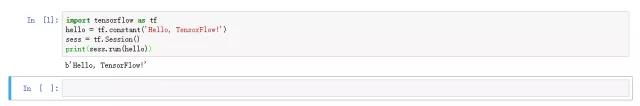TensorFlow 基础

TensorFlow 文档地址：https://www.tensorflow.org/get_started/

1.1 张量和图

TensorFlow 是一种采用数据流图（data flow graphs），用于数值计算的开源软件库。其中 Tensor 代表传递的数据为张量（多维数组），Flow 代表使用计算图进行运算。数据流图用「结点」（nodes）和「边」（edges）组成的有向图来描述数学运算。「结点」一般用来表示施加的数学操作，但也可以表示数据输入的起点和输出的终点，或者是读取/写入持久变量（persistent variable）的终点。边表示结点之间的输入/输出关系。这些数据边可以传送维度可动态调整的多维数据数组，即张量（tensor）。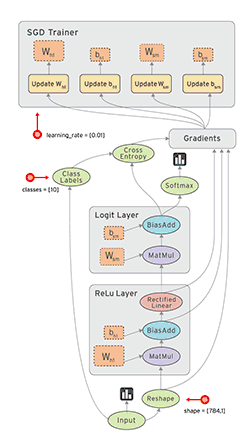1. a = tf.constant(2, tf.int16)
2. b = tf.constant(4, tf.float32)

3. graph = tf.Graph()
4. with graph.as_default():
5.    a = tf.Variable(8, tf.float32)
6.    b = tf.Variable(tf.zeros([2,2], tf.float32))

7. with tf.Session(graph=graph) as session:
8.    tf.global_variables_initializer().run()
9.    print(f)
10.    print(session.run(a))
11.    print(session.run(b))

12. #输出：
13. >>> <tf.Variable 'Variable_2:0' shape=() dtype=int32_ref>
14. >>> 8
15. >>> [[ 0.  0.]
16. >>>  [ 0.  0.]]

1. a=tf.constant([1,2],name="a")
2. b=tf.constant([2,4],name="b")
3. result = a+b
4. print(result)

1. a=tf.constant([1,2,3,4])
2. b=tf.constant([1,2,3,4])
3. result=a+b
4. sess=tf.Session()
5. print(sess.run(result))
6. sess.close

7. #输出 [2 4 6 8]

8. with tf.Session() as sess:
9.    a=tf.constant([1,2,3,4])
10.    b=tf.constant([1,2,3,4])
11.    result=a+b
12.    print(sess.run(result))

13. #输出 [2 4 6 8]

1.2 常量和变量

TensorFlow 中最基本的单位是常量（Constant）、变量（Variable）和占位符（Placeholder）。常量定义后值和维度不可变，变量定义后值可变而维度不可变。在神经网络中，变量一般可作为储存权重和其他信息的矩阵，而常量可作为储存超参数或其他结构信息的变量。下面我们分别定义了常量与变量：

1. a = tf.constant(2, tf.int16)
2. b = tf.constant(4, tf.float32)
3. c = tf.constant(8, tf.float32)

4. d = tf.Variable(2, tf.int16)
5. e = tf.Variable(4, tf.float32)
6. f = tf.Variable(8, tf.float32)

7. g = tf.constant(np.zeros(shape=(2,2), dtype=np.float32))

8. h = tf.zeros(, tf.int16)
9. i = tf.ones([2,2], tf.float32)
10. j = tf.zeros([1000,4,3], tf.float64)

11. k = tf.Variable(tf.zeros([2,2], tf.float32))
12. l = tf.Variable(tf.zeros([5,6,5], tf.float32))

w1=tf.Variable(tf.random_normal([2,3],stddev=1,seed=1))

1. weights = tf.Variable(tf.truncated_normal([256 * 256, 10]))
2. biases = tf.Variable(tf.zeros())
3. print(weights.get_shape().as_list())
4. print(biases.get_shape().as_list())

5. #输出
6. >>>[65536, 10]
7. >>>

1.3 占位符和 feed_dict

feed_dict 是一个字典，在字典中需要给出每一个用到的占位符的取值。在训练神经网络时需要每次提供一个批量的训练样本，如果每次迭代选取的数据要通过常量表示，那么 TensorFlow 的计算图会非常大。因为每增加一个常量，TensorFlow 都会在计算图中增加一个结点。所以说拥有几百万次迭代的神经网络会拥有极其庞大的计算图，而占位符却可以解决这一点，它只会拥有占位符这一个结点。

1. w1=tf.Variable(tf.random_normal([1,2],stddev=1,seed=1))

2. #因为需要重复输入x，而每建一个x就会生成一个结点，计算图的效率会低。所以使用占位符
3. x=tf.placeholder(tf.float32,shape=(1,2))
4. x1=tf.constant([[0.7,0.9]])

5. a=x+w1
6. b=x1+w1

7. sess=tf.Session()
8. sess.run(tf.global_variables_initializer())

9. #运行y时将占位符填上，feed_dict为字典，变量名不可变
10. y_1=sess.run(a,feed_dict={x:[[0.7,0.9]]})
11. y_2=sess.run(b)
12. print(y_1)
13. print(y_2)
14. sess.close

1. list_of_points1_ = [[1,2], [3,4], [5,6], [7,8]]
2. list_of_points2_ = [[15,16], [13,14], [11,12], [9,10]]

3. list_of_points1 = np.array([np.array(elem).reshape(1,2) for elem in list_of_points1_])
4. list_of_points2 = np.array([np.array(elem).reshape(1,2) for elem in list_of_points2_])

5. graph = tf.Graph()

6. with graph.as_default():

7.    #我们使用 tf.placeholder() 创建占位符 ，在 session.run() 过程中再投递数据
8.    point1 = tf.placeholder(tf.float32, shape=(1, 2))
9.    point2 = tf.placeholder(tf.float32, shape=(1, 2))

10.    def calculate_eucledian_distance(point1, point2):
11.        difference = tf.subtract(point1, point2)
12.        power2 = tf.pow(difference, tf.constant(2.0, shape=(1,2)))
15.        return eucledian_distance

16.    dist = calculate_eucledian_distance(point1, point2)

17. with tf.Session(graph=graph) as session:
18.    tf.global_variables_initializer().run()
19.    for ii in range(len(list_of_points1)):
20.        point1_ = list_of_points1[ii]
21.        point2_ = list_of_points2[ii]

22.        #使用feed_dict将数据投入到[dist]中
23.        feed_dict = {point1 : point1_, point2 : point2_}
24.        distance = session.run([dist], feed_dict=feed_dict)
25.        print("the distance between {} and {} -> {}".format(point1_, point2_, distance))

26. #输出：
27. >>> the distance between [[1 2]] and [[15 16]] -> [19.79899]
28. >>> the distance between [[3 4]] and [[13 14]] -> [14.142136]
29. >>> the distance between [[5 6]] and [[11 12]] -> [8.485281]
30. >>> the distance between [[7 8]] and [[ 9 10]] -> [2.8284271]

Ahmet Taspinar 在第二部分就直接开始构建深度神经网络了，虽然我们在前一章增加了许多代码段以帮助读者了解 TensorFlow 的基本法则，但上面是远远不够的。所以如果我们能先解析一部分神经网络代码，那么将有助于入门读者巩固以上的 TensorFlow 基本知识。下面，我们将先解析一段构建了三层全连接神经网络的代码。

1. import tensorflow as tf
2. from numpy.random import RandomState

3. batch_size=10
4. w1=tf.Variable(tf.random_normal([2,3],stddev=1,seed=1))
5. w2=tf.Variable(tf.random_normal([3,1],stddev=1,seed=1))

6. # None 可以根据batch 大小确定维度，在shape的一个维度上使用None
7. x=tf.placeholder(tf.float32,shape=(None,2))
8. y=tf.placeholder(tf.float32,shape=(None,1))

9. #激活函数使用ReLU
10. a=tf.nn.relu(tf.matmul(x,w1))
11. yhat=tf.nn.relu(tf.matmul(a,w2))

13. cross_entropy=-tf.reduce_mean(y*tf.log(tf.clip_by_value(yhat,1e-10,1.0)))

15. rdm=RandomState(1)
16. data_size=516

17. #生成两个特征，共data_size个样本
18. X=rdm.rand(data_size,2)
19. #定义规则给出样本标签，所有x1+x2<1的样本认为是正样本，其他为负样本。Y，1为正样本
20. Y = [[int(x1+x2 < 1)] for (x1, x2) in X]

21. with tf.Session() as sess:
22.    sess.run(tf.global_variables_initializer())
23.    print(sess.run(w1))
24.    print(sess.run(w2))
25.    steps=11000
26.    for i in range(steps):

27.        #选定每一个批量读取的首尾位置，确保在1个epoch内采样训练
28.        start = i * batch_size % data_size
29.        end = min(start + batch_size,data_size)
30.        sess.run(train_step,feed_dict={x:X[start:end],y:Y[start:end]})
31.        if i % 1000 == 0:
32.            training_loss= sess.run(cross_entropy,feed_dict={x:X,y:Y})
33.            print("在迭代 %d 次后，训练损失为 %g"%(i,training_loss))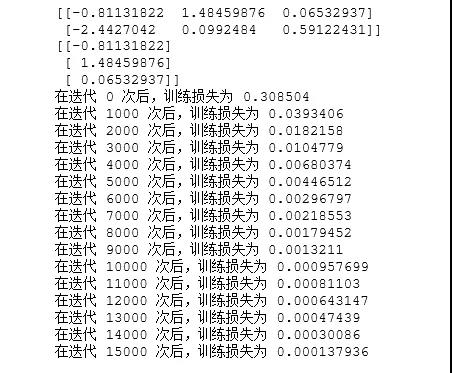TensorFlow 中的神经网络

2.1 简介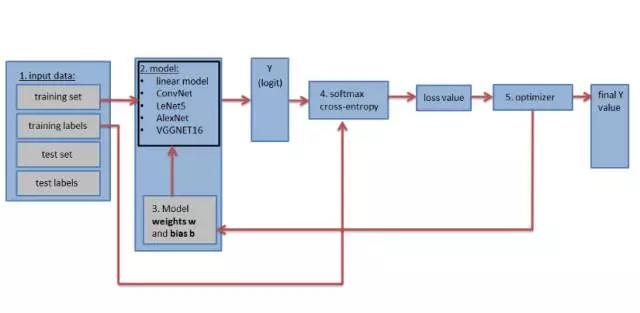• 输入数据集，数据集分为训练数据集和标注、测试数据集和标注（包括验证数据集和标注）。测试和验证集能赋值到 tf.constant() 中，而训练集可以导入 tf.placeholder() 中，训练集只有导入占位符我们才能在随机梯度下降中成批量地进行训练。
• 确定神经网络模型，该模型可以是简单的一层全连接网络或 9 层、16 层的复杂卷积网络组成。
• 网络定义的权重矩阵和偏置向量后需要执行初始化，每一层需要一个权重矩阵和一个偏置向量。
• 构建损失函数，并计算训练损失。模型会输出一个预测向量，我们可以比较预测标签和真实标签并使用交叉熵函数和 softmax 回归来确定损失值。训练损失衡量预测值和真实值之间差距，并用于更新权重矩阵。
• 优化器，优化器将使用计算的损失值和反向传播算法更新权重和偏置项参数。

2.2 加载数据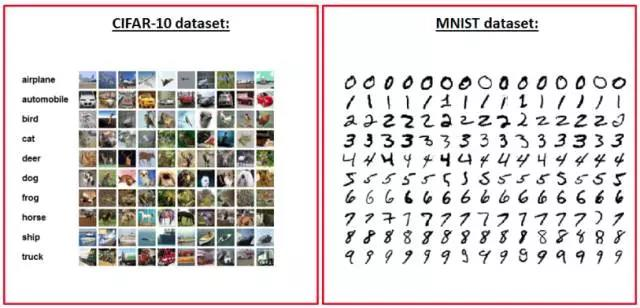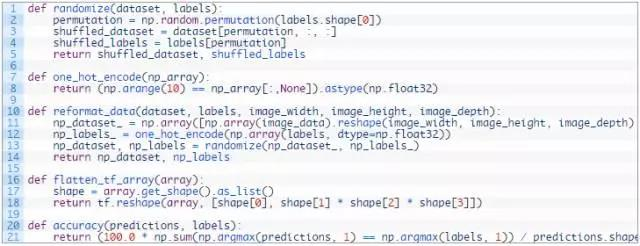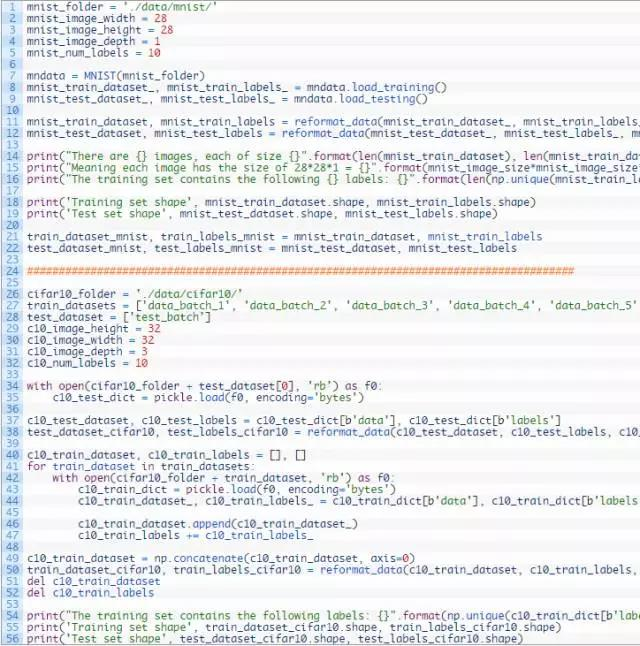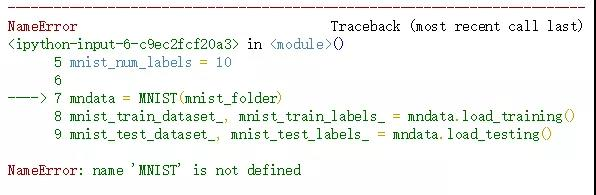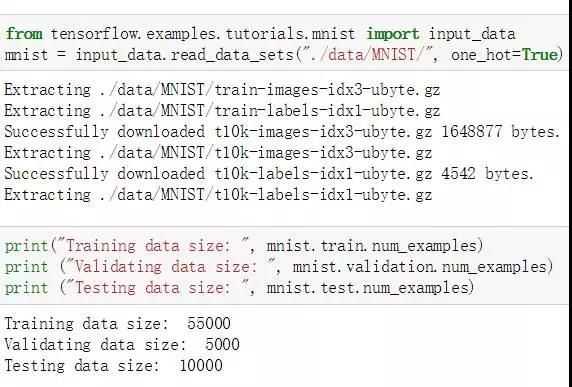1. cifar10_folder = './data/cifar10/'
2. train_datasets = ['data_batch_1', 'data_batch_2', 'data_batch_3', 'data_batch_4', 'data_batch_5', ]
3. test_dataset = ['test_batch']

4. c10_image_height = 32
5. c10_image_width = 32
6. c10_image_depth = 3
7. c10_num_labels = 10
8. c10_image_size = 32 #Ahmet Taspinar的代码缺少了这一语句

9. with open(cifar10_folder + test_dataset, 'rb') as f0:

11. c10_test_dataset, c10_test_labels = c10_test_dict[b'data'], c10_test_dict[b'labels']
12. test_dataset_cifar10, test_labels_cifar10 = reformat_data(c10_test_dataset, c10_test_labels, c10_image_size, c10_image_size, c10_image_depth)

13. c10_train_dataset, c10_train_labels = [], []

14. for train_dataset in train_datasets:
15.    with open(cifar10_folder + train_dataset, 'rb') as f0:
17.        c10_train_dataset_, c10_train_labels_ = c10_train_dict[b'data'], c10_train_dict[b'labels']

18.        c10_train_dataset.append(c10_train_dataset_)
19.        c10_train_labels += c10_train_labels_

20. c10_train_dataset = np.concatenate(c10_train_dataset, axis=0)
21. train_dataset_cifar10, train_labels_cifar10 = reformat_data(c10_train_dataset, c10_train_labels, c10_image_size, c10_image_size, c10_image_depth)
22. del c10_train_dataset
23. del c10_train_labels

24. print("训练集包含以下标签: {}".format(np.unique(c10_train_dict[b'labels'])))
25. print('训练集维度', train_dataset_cifar10.shape, train_labels_cifar10.shape)
26. print('测试集维度', test_dataset_cifar10.shape, test_labels_cifar10.shape)

2.3 创建简单的多层全连接神经网络

Ahmet Taspinar 后面创建了一个单隐藏层全连接网络，不过我们还是报错了。他在博客中给出了以下训练准确度，我们看到该模型在 MNIST 数据集效果并不是很好。所以我们另外使用一个全连接神经网络来实现这一过程。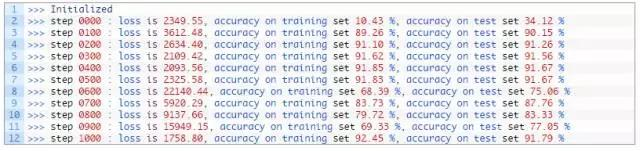1. import tensorflow as tf
2. from tensorflow.examples.tutorials.mnist import input_data

3. #加载MNIST数据集

5. INPUT_NODE = 784
6. OUTPUT_NODE = 10
7. LAYER1_NODE = 500

8. BATCH_SIZE = 100

9.

10. # 模型相关的参数
11. LEARNING_RATE_BASE = 0.8
12. LEARNING_RATE_DECAY = 0.99
13. REGULARAZTION_RATE = 0.0001
14. TRAINING_STEPS = 10000
15. MOVING_AVERAGE_DECAY = 0.99

16. def inference(input_tensor, avg_class, weights1, biases1, weights2, biases2):
17.    # 使用滑动平均类
18.    if avg_class == None:
19.        layer1 = tf.nn.relu(tf.matmul(input_tensor, weights1) + biases1)
20.        return tf.matmul(layer1, weights2) + biases2

21.    else:

22.        layer1 = tf.nn.relu(tf.matmul(input_tensor, avg_class.average(weights1)) + avg_class.average(biases1))
23.        return tf.matmul(layer1, avg_class.average(weights2)) + avg_class.average(biases2)

24. def train(mnist):
25.    x = tf.placeholder(tf.float32, [None, INPUT_NODE], name='x-input')
26.    y_ = tf.placeholder(tf.float32, [None, OUTPUT_NODE], name='y-input')

27.    # 生成隐藏层的参数。
28.    weights1 = tf.Variable(tf.truncated_normal([INPUT_NODE, LAYER1_NODE], stddev=0.1))
29.    biases1 = tf.Variable(tf.constant(0.1, shape=[LAYER1_NODE]))

30.    # 生成输出层的参数。
31.    weights2 = tf.Variable(tf.truncated_normal([LAYER1_NODE, OUTPUT_NODE], stddev=0.1))
32.    biases2 = tf.Variable(tf.constant(0.1, shape=[OUTPUT_NODE]))

33.    # 计算不含滑动平均类的前向传播结果
34.    y = inference(x, None, weights1, biases1, weights2, biases2)

35.    # 定义训练轮数及相关的滑动平均类
36.    global_step = tf.Variable(0, trainable=False)
37.    variable_averages = tf.train.ExponentialMovingAverage(MOVING_AVERAGE_DECAY, global_step)
38.    variables_averages_op = variable_averages.apply(tf.trainable_variables())
39.    average_y = inference(x, variable_averages, weights1, biases1, weights2, biases2)

40.    # 计算交叉熵及其平均值
41.    cross_entropy = tf.nn.sparse_softmax_cross_entropy_with_logits(logits=y, labels=tf.argmax(y_, 1))
42.    cross_entropy_mean = tf.reduce_mean(cross_entropy)

43.    # 定义交叉熵损失函数加上正则项为模型损失函数
44.    regularizer = tf.contrib.layers.l2_regularizer(REGULARAZTION_RATE)
45.    regularaztion = regularizer(weights1) + regularizer(weights2)
46.    loss = cross_entropy_mean + regularaztion

47.    # 设置指数衰减的学习率。
48.    learning_rate = tf.train.exponential_decay(
49.        LEARNING_RATE_BASE,
50.        global_step,
51.        mnist.train.num_examples / BATCH_SIZE,
52.        LEARNING_RATE_DECAY,
53.        staircase=True)

54.    # 随机梯度下降优化器优化损失函数

56.    # 反向传播更新参数和更新每一个参数的滑动平均值
57.    with tf.control_dependencies([train_step, variables_averages_op]):
58.        train_op = tf.no_op(name='train')

59.    # 计算准确度
60.    correct_prediction = tf.equal(tf.argmax(average_y, 1), tf.argmax(y_, 1))
61.    accuracy = tf.reduce_mean(tf.cast(correct_prediction, tf.float32))

62.    # 初始化会话并开始训练过程。
63.    with tf.Session() as sess:
64.        tf.global_variables_initializer().run()
65.        validate_feed = {x: mnist.validation.images, y_: mnist.validation.labels}
66.        test_feed = {x: mnist.test.images, y_: mnist.test.labels}

67.        # 循环地训练神经网络。
68.        for i in range(TRAINING_STEPS):
69.            if i % 1000 == 0:
70.                validate_acc = sess.run(accuracy, feed_dict=validate_feed)
71.                print("After %d training step(s), validation accuracy using average model is %g " % (i, validate_acc))

72.            xs,ys=mnist.train.next_batch(BATCH_SIZE)
73.            sess.run(train_op,feed_dict={x:xs,y_:ys})

74.        test_acc=sess.run(accuracy,feed_dict=test_feed)
75.        print(("After %d training step(s), test accuracy using average model is %g" %(TRAINING_STEPS, test_acc)))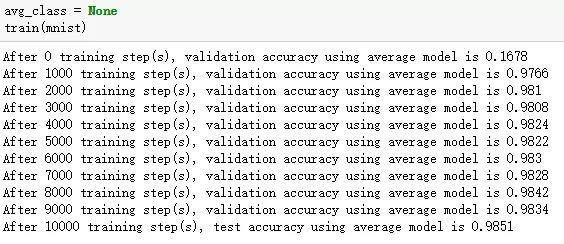TensorFlow 包含很多操作和函数，很多我们需要花费大量精力完成的过程可以直接调用已封装的函数，比如说「logits = tf.matmul(tf_train_dataset, weights) + biases」可以由函数「logits = tf.nn.xw_plus_b(train_dataset, weights, biases)」代替。

1. import tensorflow as tf

2. w1 = tf.Variable(tf.truncated_normal([filter_size, filter_size, image_depth, filter_depth], stddev=0.1))
3. b1 = tf.Variable(tf.zeros([filter_depth]))

4. layer1_conv = tf.nn.conv2d(data, w1, [1, 1, 1, 1], padding='SAME')
5. layer1_relu = tf.nn.relu(layer1_conv + b1)
6. layer1_pool = tf.nn.max_pool(layer1_pool, [1, 2, 2, 1], [1, 2, 2, 1], padding='SAME')

1. from tflearn.layers.conv import conv_2d, max_pool_2d

2. layer1_conv = conv_2d(data, filter_depth, filter_size, activation='relu')
3. layer1_pool = max_pool_2d(layer1_conv_relu, 2, strides=2)

2.4 创建 LeNet5 卷积网络

LeNet5 卷积网络架构最早是 Yann LeCun 提出来的，它是早期的一种卷积神经网络，并且可以用来识别手写数字。虽然它在 MNIST 数据集上执行地非常好，但在其它高分辨率和大数据集上性能有所降低。对于这些大数据集，像 AlexNet、VGGNet 或 ResNet 那样的深度卷积网络才执行地十分优秀。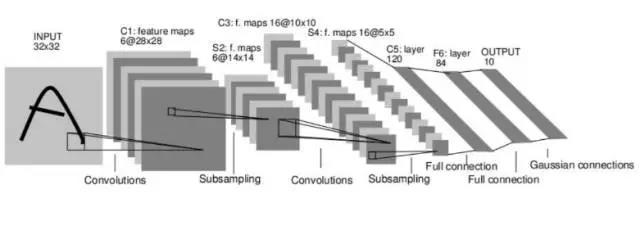LeNet5 包含 5 层网络：

• 第一层：卷积层，该卷积层使用 Sigmoid 激活函数，并且在后面带有平均池化层。
• 第二层：卷积层，该卷积层使用 Sigmoid 激活函数，并且在后面带有平均池化层。
• 第三层：全连接层（使用 Sigmoid 激活函数）。
• 第四层：全连接层（使用 Sigmoid 激活函数）。
• 第五层：输出层。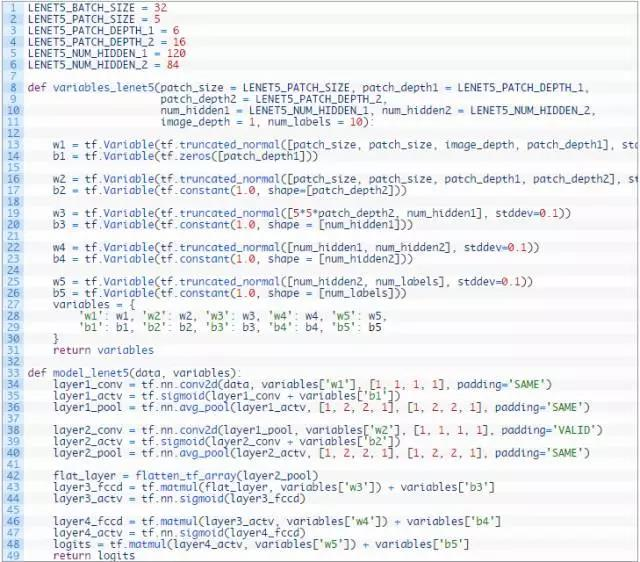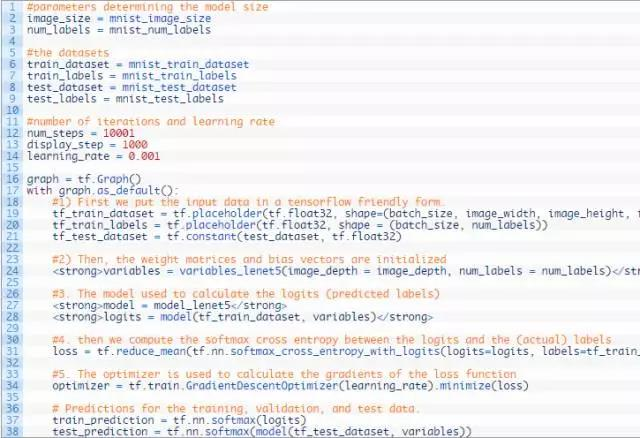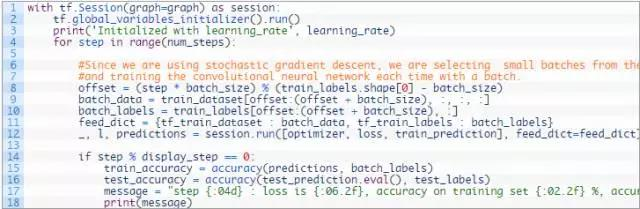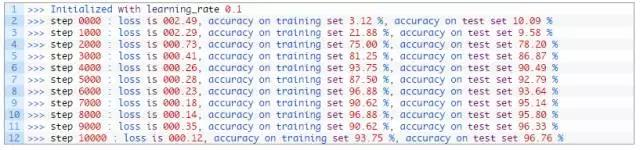2.5 超参数如何影响一层网络的输出尺寸

• 输入图像，即一个四维张量 [batch size, image_width, image_height, image_depth]
• 权重矩阵，即一个四维张量 [filter_size, filter_size, image_depth, filter_depth]
• 每一个维度的步幅数
• Padding (= 'SAME' / 'VALID')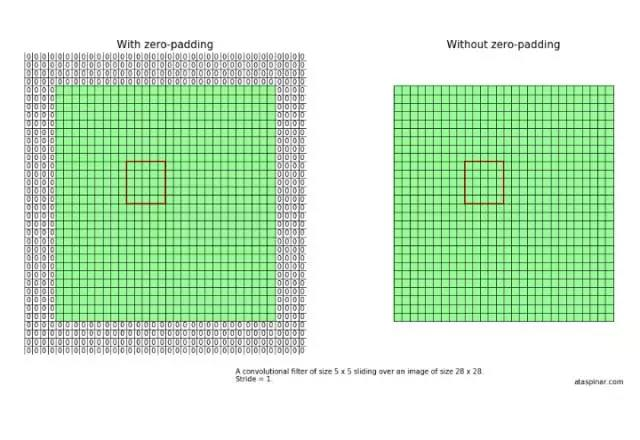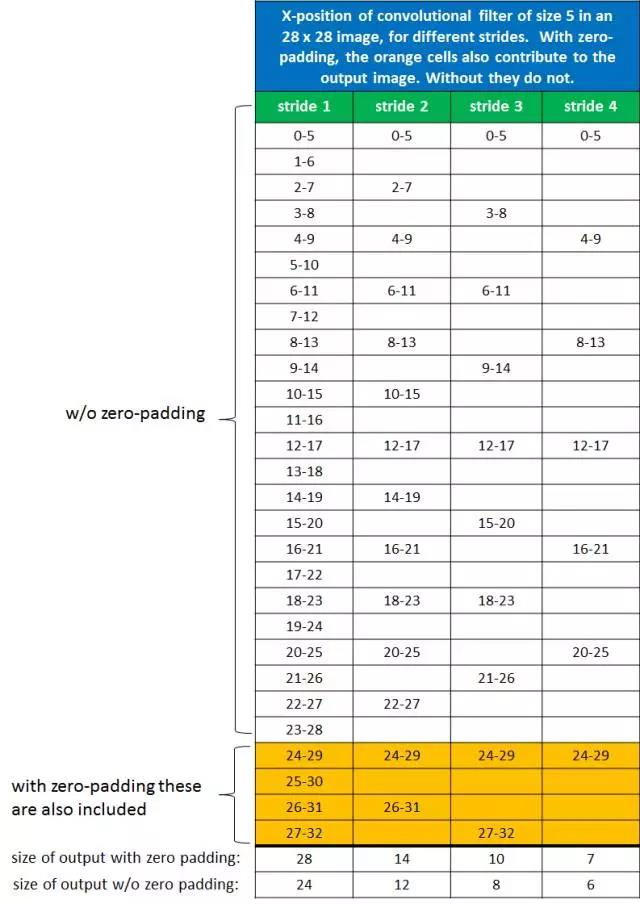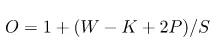2.6 调整 LeNet5 架构

LeNet5 架构在原论文中使用的是 Sigmoid 激活函数和平均池化。然而如今神经网络使用 ReLU 激活函数更为常见。所以我们可以修改一下 LeNet5 架构，并看看是否能获得性能上的提升，我们可以称这种修改的架构为类 LeNet5 架构。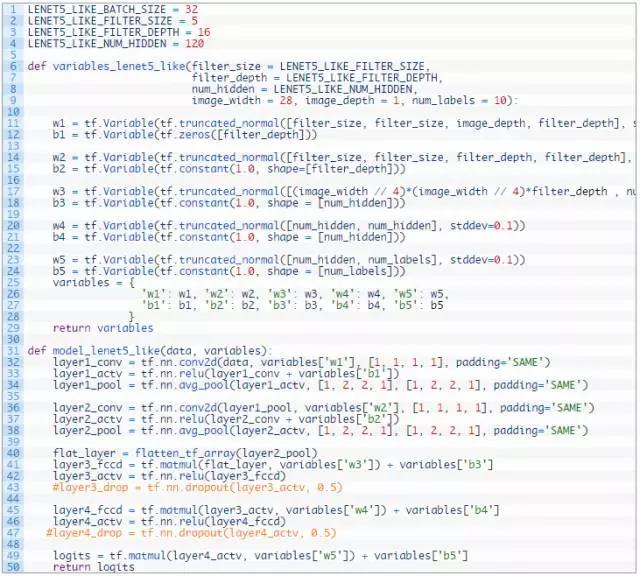1. LENET5_LIKE_BATCH_SIZE = 32
2. LENET5_LIKE_FILTER_SIZE = 5
3. LENET5_LIKE_FILTER_DEPTH = 16
4. LENET5_LIKE_NUM_HIDDEN = 120

5. def variables_lenet5_like(filter_size = LENET5_LIKE_FILTER_SIZE,
6.                          filter_depth = LENET5_LIKE_FILTER_DEPTH,
7.                          num_hidden = LENET5_LIKE_NUM_HIDDEN,
8.                          image_width = 32, image_height = 32, image_depth = 3, num_labels = 10):

9.    w1 = tf.Variable(tf.truncated_normal([filter_size, filter_size, image_depth, filter_depth], stddev=0.1))
10.    b1 = tf.Variable(tf.zeros([filter_depth]))

11.    w2 = tf.Variable(tf.truncated_normal([filter_size, filter_size, filter_depth, filter_depth], stddev=0.1))
12.    b2 = tf.Variable(tf.constant(1.0, shape=[filter_depth]))

13.    w3 = tf.Variable(tf.truncated_normal([(image_width // 4)*(image_height // 4)*filter_depth , num_hidden], stddev=0.1))
14.    b3 = tf.Variable(tf.constant(1.0, shape = [num_hidden]))

15.    w4 = tf.Variable(tf.truncated_normal([num_hidden, num_hidden], stddev=0.1))
16.    b4 = tf.Variable(tf.constant(1.0, shape = [num_hidden]))

17.    w5 = tf.Variable(tf.truncated_normal([num_hidden, num_labels], stddev=0.1))
18.    b5 = tf.Variable(tf.constant(1.0, shape = [num_labels]))
19.    variables = {
20.        'w1': w1, 'w2': w2, 'w3': w3, 'w4': w4, 'w5': w5,
21.        'b1': b1, 'b2': b2, 'b3': b3, 'b4': b4, 'b5': b5
22.    }
23.    return variables

24. def model_lenet5_like(data, variables):
25.    layer1_conv = tf.nn.conv2d(data, variables['w1'], [1, 1, 1, 1], padding='SAME')
26.    layer1_actv = tf.nn.relu(layer1_conv + variables['b1'])
27.    layer1_pool = tf.nn.avg_pool(layer1_actv, [1, 2, 2, 1], [1, 2, 2, 1], padding='SAME')

28.    layer2_conv = tf.nn.conv2d(layer1_pool, variables['w2'], [1, 1, 1, 1], padding='SAME')
29.    layer2_actv = tf.nn.relu(layer2_conv + variables['b2'])
30.    layer2_pool = tf.nn.avg_pool(layer2_actv, [1, 2, 2, 1], [1, 2, 2, 1], padding='SAME')

31.    flat_layer = flatten_tf_array(layer2_pool)
32.    layer3_fccd = tf.matmul(flat_layer, variables['w3']) + variables['b3']
33.    layer3_actv = tf.nn.relu(layer3_fccd)
34.    layer3_drop = tf.nn.dropout(layer3_actv, 0.5)

35.    layer4_fccd = tf.matmul(layer3_actv, variables['w4']) + variables['b4']
36.    layer4_actv = tf.nn.relu(layer4_fccd)
37.    layer4_drop = tf.nn.dropout(layer4_actv, 0.5)

38.    logits = tf.matmul(layer4_actv, variables['w5']) + variables['b5']
39.    return logits

40. num_steps = 10001
41. display_step = 1000
42. learning_rate = 0.001
43. batch_size = 16

44. #定义数据的基本信息，传入变量
45. image_width = 32
46. image_height = 32
47. image_depth = 3
48. num_labels = 10

49. test_dataset = test_dataset_cifar10
50. test_labels = test_labels_cifar10
51. train_dataset = train_dataset_cifar10
52. train_labels = train_labels_cifar10

53. graph = tf.Graph()

54. with graph.as_default():

55.    #1 首先使用占位符定义数据变量的维度
56.    tf_train_dataset = tf.placeholder(tf.float32, shape=(batch_size, image_width, image_height, image_depth))
57.    tf_train_labels = tf.placeholder(tf.float32, shape = (batch_size, num_labels))
58.    tf_test_dataset = tf.constant(test_dataset, tf.float32)

59.    #2 然后初始化权重矩阵和偏置向量
60.    variables = variables_lenet5_like(image_width = image_width, image_height=image_height, image_depth = image_depth, num_labels = num_labels)

61.    #3 使用模型计算分类
62.    logits = model_lenet5_like(tf_train_dataset, variables)

63.    #4 使用带softmax的交叉熵函数计算预测标签和真实标签之间的损失函数
64.    loss = tf.reduce_mean(tf.nn.softmax_cross_entropy_with_logits(logits=logits, labels=tf_train_labels))

67.

# 执行预测推断

68.    train_prediction = tf.nn.softmax(logits)
69.    test_prediction = tf.nn.softmax(model_lenet5_like(tf_test_dataset, variables))

70. with tf.Session(graph=graph) as session:

71.    #初始化全部变量
72.    tf.global_variables_initializer().run()
73.    print('Initialized with learning_rate', learning_rate)
74.    for step in range(num_steps):
75.        offset = (step * batch_size) % (train_labels.shape - batch_size)
76.        batch_data = train_dataset[offset:(offset + batch_size), :, :, :]
77.        batch_labels = train_labels[offset:(offset + batch_size), :]

78.        #在每一次批量中，获取当前的训练数据，并传入feed_dict以馈送到占位符中
79.        feed_dict = {tf_train_dataset : batch_data, tf_train_labels : batch_labels}
80.        _, l, predictions = session.run([optimizer, loss, train_prediction], feed_dict=feed_dict)
81.        train_accuracy = accuracy(predictions, batch_labels)

82.        if step % display_step == 0:
83.            test_accuracy = accuracy(test_prediction.eval(), test_labels)
84.            message = "step {:04d} : loss is {:06.2f}, accuracy on training set {:02.2f} %, accuracy on test set {:02.2f} %".format(step, l, train_accuracy, test_accuracy)
85.            print(message)

2.7 学习率和优化器的影响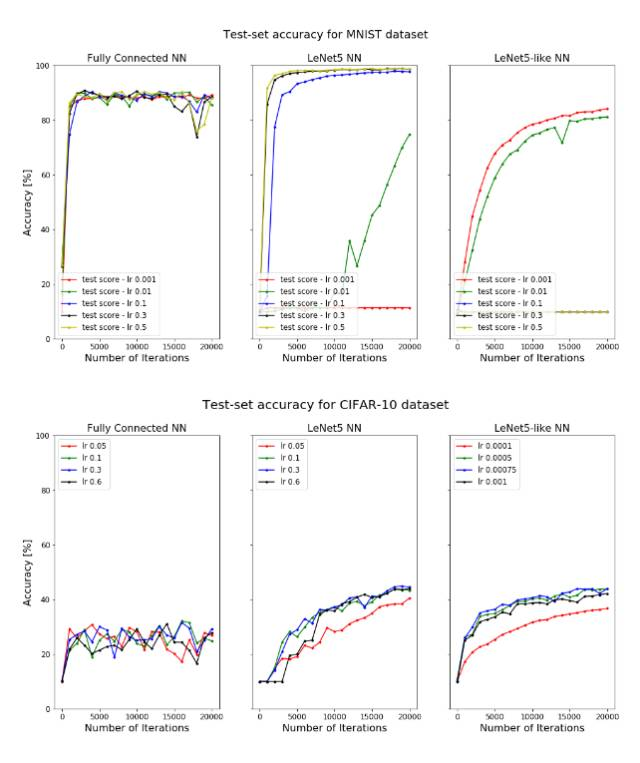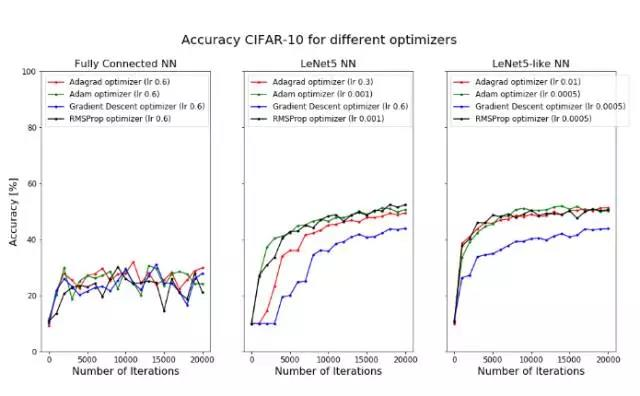TensorFlow 中的深度神经网络

LeNet5 由两个卷积层加上三个全连接层组成，因此它是一种浅层神经网络。下面我们将了解其它卷积神经网络，它们的层级更多，所以可以称为深度神经网络。下面介绍的深度卷积神经网络我们并没有根据 Ahmet Taspinar 提供的代码进行实践，因为我们暂时安装的是 TensorFlow 的 CPU 版，而使用 CPU 训练前面的 LeNet 就已经十分吃力了，所以我们暂时没有实现这几个深度 CNN。我们将会在后面实现它们，并将修改的代码上传到机器之心的 Github 中。

3.1 AlexNet

AlexNet 是由 Alex Krizhevsky 和 Geoffrey Hinton 等人提出来的，虽然相对于现在的卷积神经网络来说它的架构十分简单，但当时它是十分成功的一个模型。它赢得了当年的 ImageNet 挑战赛，并开启了深度学习和 AI 的变革。下面是 AlexNet 的基本架构：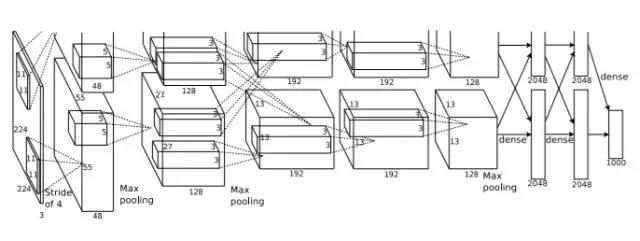AlexNet 包含 5 个卷积层（带有 ReLU 激活函数）、3 个最大池化层、3 个全连接层和两个 dropout 层。该神经网络的架构概览如下：

• 层级 0：规格为 224 x 224 x 3 的输入图片。
• 层级 1：带有 96 个滤波器（filter_depth_1 = 96）的卷积层，滤波器的尺寸为 11 x 11（filter_size_1 = 11）、步幅为 4。该层的神经网络使用 ReLU 激活函数，并且后面带有最大池化层和局部响应归一化层。
• 层级 2：带有 256 个滤波器（filter_depth_2 = 256）的卷积层，滤波器的尺寸为 5 x 5（filter_size_2 = 5）、步幅为 1。该层的神经网络使用 ReLU 激活函数，并且后面带有最大池化层和局部响应归一化层。
• 层级 3：带有 384 个滤波器（filter_depth_3 = 384）的卷积层，滤波器的尺寸为 3 x 3（filter_size_3 = 3）、步幅为 1。该层的神经网络使用 ReLU 激活函数。
• 层级 4 和层级 3 的结构是一样的。
• 层级 5：带有 256 个滤波器（filter_depth_4 = 256）的卷积层，滤波器的尺寸为 3 x 3（filter_size_4 = 3）、步幅为 1。该层的神经网络使用 ReLU 激活函数。
• 层级 6-8：这几个卷积层每一个后面跟着一个全连接层，每一层有 4096 个神经元。在原论文中，他们是为了 1000 个类别的分类，当我们这边并不需要这么多。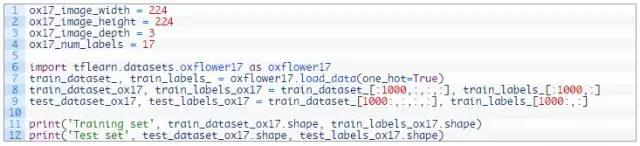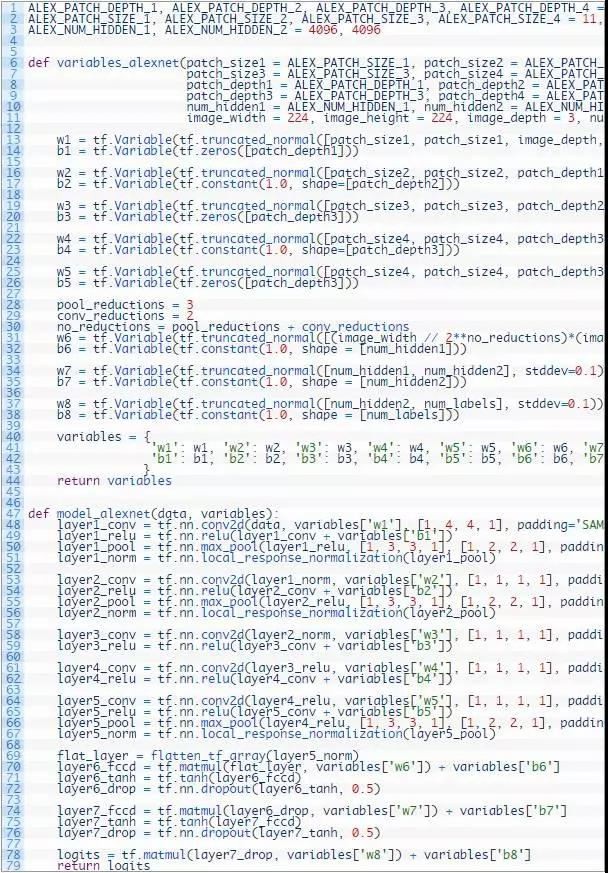3.2 VGGNet-16

VGGNet 比 AlexNet 拥有的层级更多（16-19 层），但是每一层的设计都简单了许多，所有层的滤波器大小都是 3 x 3、步幅都是 1，而所有的最大池化层的步幅都是 2。所以它虽然是一种深度 CNN，但结构比较简单。

VGGNet 有 16 层或 19 层两种配置，如下所示，这两种配置的不同之处在于它在第二个、第三个和第四个最大池化层后面到底是采用三个卷积层还是四个卷积层。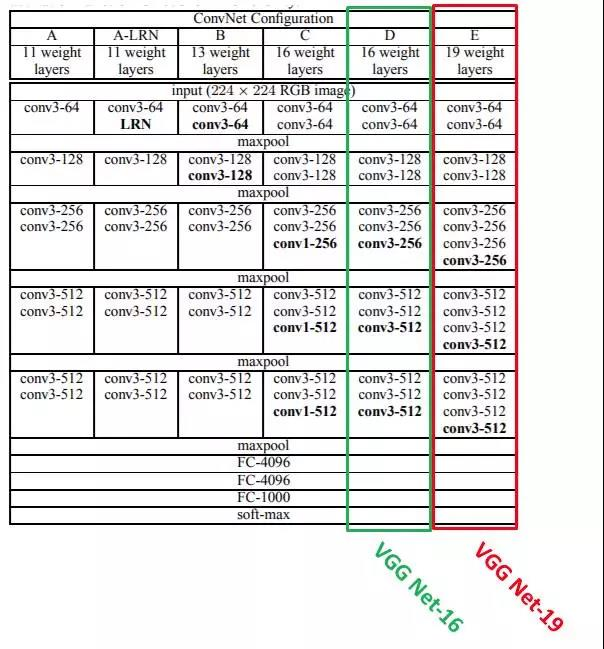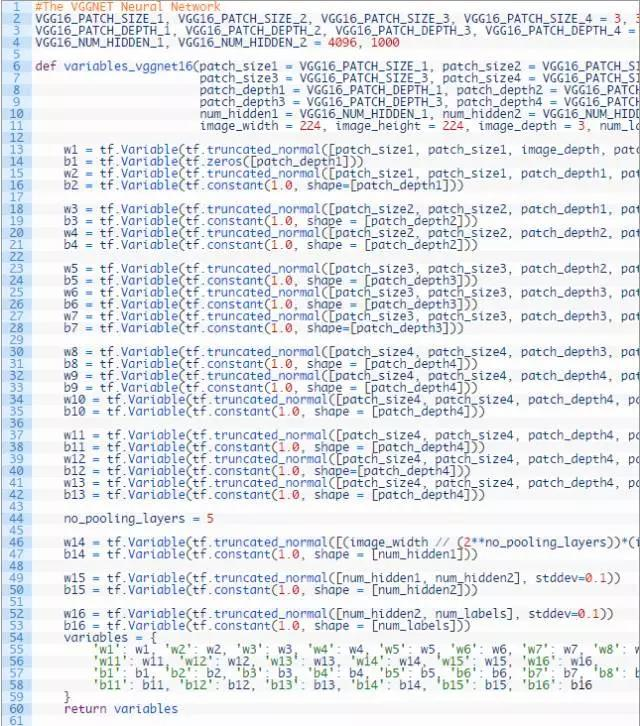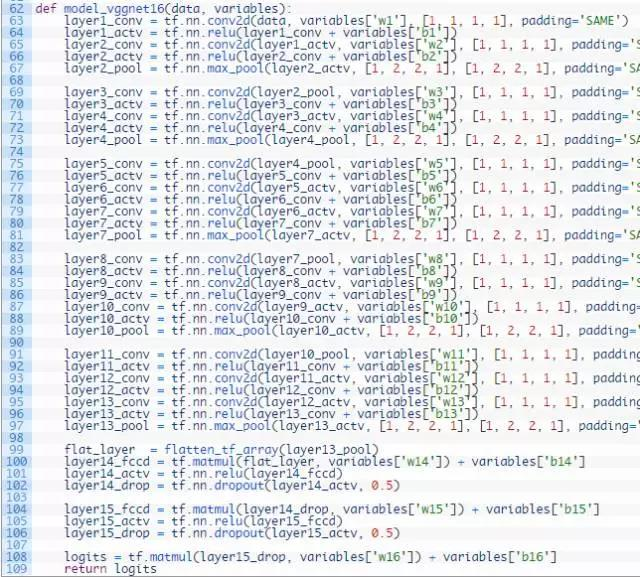【项目积累】对JSON数据的处理
<p><span style="font-family:楷体; font-family:楷体; font-size:19px; line-height:27px; widows:auto">   <strong>【项目简述】</strong></span><strong><span style="font-family:楷体; font-family:楷体; font-size:19px;
2842 0GitHub上10个最受欢迎的安全项目
1652 0Github 开源项目（二） jsmpeg-vnc

1252 02015 年，哪些开源项目荣登 GitHub 十强榜单？
1710 01032 0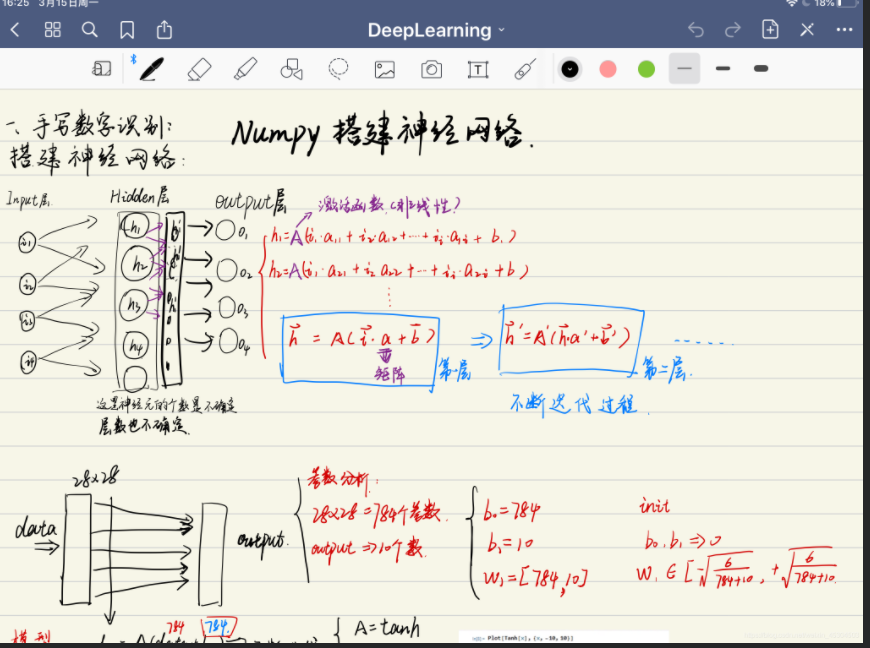​ 利用纯numpy实现手势识别，首先是进行的整体的网络构成，然后再展示代码部分。这是我的第一个神经网络。 完整代码： GitHub 网络大体体现： ​ 输入层，隐藏层，输出层。已经知道的是输出层是有十个结果的，就是10个数字的概率。
21 016633 0Eclipse中的创建maven项目，添加src/main/java等source folder
1228 016 0
1946

0

《2021云上架构与运维峰会演讲合集》

《零基础CSS入门教程》

《零基础HTML入门教程》